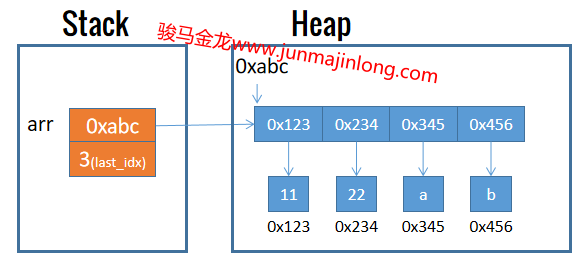## 数组的基本使用

``````my @arr = (11, 22, 33, 44);  # 只包含数值的数组
``````

Perl数组是动态的，不限制数组的长度(元素个数)，可以在任何时候往数组中增加任意多的元素。例如可以使用push函数向数组中追加元素。

``````my @arr = ();   # 声明空数组
push @arr, 11;
push @arr, 22;
say "@arr";  # 别忘了，双引号允许内插数组
# 输出：11 22
``````

Perl数组也不限制所存放元素的数据类型。例如：

``````# 数组中同时保存数值、字符串
my @arr = (11,22,'a','b');
``````### qw创建数组

Perl提供了类似于q和qq的qw字面量表示法，qw的全称是quote words，即自动引用单词。perl会在编译期间将qw所引用的单词自动替换为单引号。

``````my @arr1 = ('a', 'b', 'c', 'd');
my @arr2 = qw(a b c d);
``````

``````qw()
qw[]
qw!!
qw%%
``````

### 索引数组

``````my @arr = (11,22,33,44);
# 读取index=0位置处也就是第一个元素
say \$arr;
``````

Perl还支持负数索引，表示从数组尾部开始计算元素的位置。index=-1表示倒数第一个也就是最后一个元素的位置，index=-2表示倒数第二个元素的位置。

``````my @arr = (11,22,33,44);
say \$arr[-1];     # 44
say \$arr[-2];     # 33
``````

``````my @arr = (11,22,33,44);
# 作为左值被赋值
\$arr = 222;
\$arr[-1] = 444;
say "@arr";   # 输出：11 222 33 444
``````

``````my @arr = (11,22,33,44);
say \$arr;     # 输出空字符串，数组不变

# 数组长度为11，最后一个索引为index=10
# 中间index=4到index=9的元素都被填充为undef
\$arr = 9999;
``````

### 扩展数组

``````my @arr = (11,22,33);
@arr = (@arr, 44, 55);  # 扩展数组
say "@arr";  # 11 22 33 44 55
``````

``````my @arr = (11,22,33);
# 数组先展开，得到(11,22,33)，然后赋值给@arr1
my @arr1 = (@arr);
``````

### 数组长度

• 数组转换为标量得到的是数组的长度
• hash转换为标量得到的是`M/N`格式的字符串，N表示hash的容量，M表示hash当前的键值对数量

``````my @arr = (11,22,33,44);
my %p = (
name => "junmajinlong",
age => 23,
gender => "male",
);

# scalar使其参数强制进入标量上下文
say scalar @arr;    # 输出：4
say scalar %p;      # 输出：3/8
``````

``````my @arr = (11,22,33);
\$arr[scalar @arr] = 44;
say "@arr";  # 输出：11 22 33 44
``````

### 数组最大索引

``````my @arr = (11, 22, 33, 44);
my \$arr_max_idx = scalar @arr - 1;  # 3
``````

``````my @arr = (11, 22, 33, 44);
say \$#arr;   # 3
``````

``````my @arr = (11,22,33);
\$arr[++\$#arr] = 44;
say "@arr";  # 输出：11 22 33 44
``````

``````my @arr = (11,22,33,44);
\$#arr = 10; # 将修改长度为11，缺失元素使用undef填充
\$#arr = 2;  # 将长度修改为3，截断元素44和后面的空元素
``````

### 字符串内插数组

``````my @arr = (11, 22, 33);

# 内插后，得到字符串："arr: 11 22 33"
my \$str = "arr: @arr";
``````

``````say qq(x\${"}x);  # 输出： x x
``````

``````my @arr = (11, 22, 33, 44);
\$" = "-";
say "@arr";   # 11-22-33-44
``````

### print/say输出数组

``````my @arr = (11, 22, 33);
say @arr;  # 输出：112233
``````

``````my @arr = (11, 22, 33);

# 11 22 33 a b共5个元素共同组成一个列表
say @arr,'a','b';
``````

print/say输出时，默认使用内置变量`\$,`来分隔列表各元素，该内置变量默认值为undef，因此默认情况下各元素被输出时紧密相连。

``````my @arr = (11, 22, 33);
say @arr,'a','b';  # 112233ab

\$, = "_";   # 将其修改为下划线
say @arr,'a','b';  # 11_22_33_a_b
``````